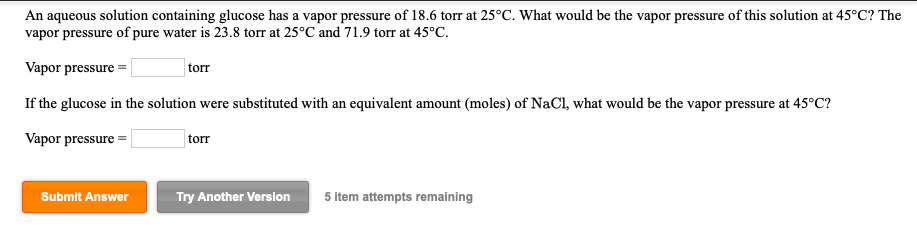# An aqueous solution containing glucose has a vapor pressure of 18.6 torr at 25°C. What would be the vapor pressure of this solution at 45°C? Thevapor pressure of pure water is 23.8 torr at 25 °C and 71.9 torr at 45°C.Vapor pressureIf the glucose in the solution were substituted with an equivalent amount (moles) of NaCl, what would be the vapor pressure at 45°C?Vapor pressuretorrtorrSubmit AnswerTry Another Version5 item attempts remaining

Question
166 viewshelp_outlineImage TranscriptioncloseAn aqueous solution containing glucose has a vapor pressure of 18.6 torr at 25°C. What would be the vapor pressure of this solution at 45°C? The vapor pressure of pure water is 23.8 torr at 25 °C and 71.9 torr at 45°C. Vapor pressure If the glucose in the solution were substituted with an equivalent amount (moles) of NaCl, what would be the vapor pressure at 45°C? Vapor pressure torr torr Submit Answer Try Another Version 5 item attempts remaining fullscreen
check_circle

Step 1

Let P0 be the vapor pressure of the pure solvent and p be the vapor pressure of solution.

i be the van't Hoff factor

x be the mole fraction of the solute.

The expression for relative lowering of pressure is:

Step 2

At 250C, for a solution of glucose in water,

P0=23.8 torr

p=18.6 torr

Since glucose does not dissociate or associate in water, so i=1

Substituting the values to get mole fraction of glucose :

Step 3

At 450C for a solution of glu...

### Want to see the full answer?

See Solution

#### Want to see this answer and more?

Solutions are written by subject experts who are available 24/7. Questions are typically answered within 1 hour.*

See Solution
*Response times may vary by subject and question.
Tagged in

### Solutions